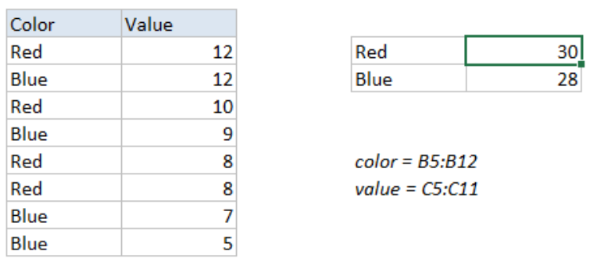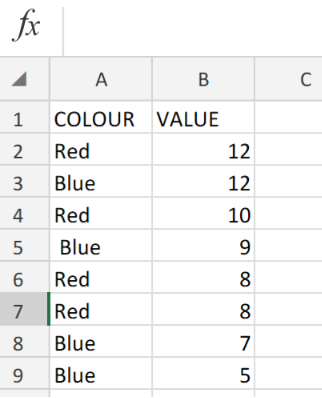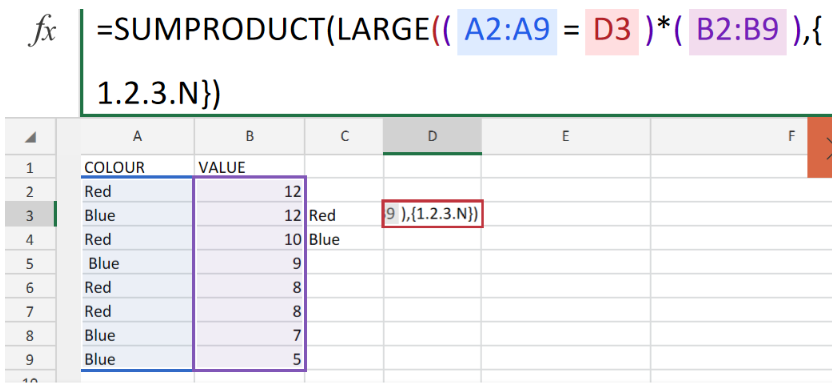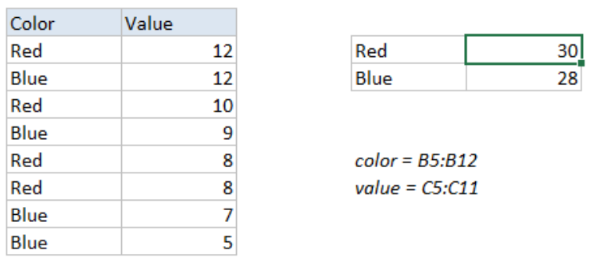Get instant live expert help with Excel or Google Sheets“My Excelchat expert helped me in less than 20 minutes, saving me what would have been 5 hours of work!”

#### Post your problem and you’ll get Expert help in seconds.

Your message must be at least 40 characters
Our professional Expert are available now. Your privacy is guaranteed.

# Sum Top n Values with CriteriaFigure 1. of Sum Top Values with Criteria.

In order to get the sum of the top n values within an array of matching data values, we are going to utilize a formula which is derived from the Excel LARGE Function, embedded within the Excel SUMPRODUCT Function.

## Generic Formula

`=SUMPRODUCT(LARGE((range=criteria)*(values),{1,2,3,N}))`

Where range = array of cells in our worksheet that matches with specific criteria and values = number values which contain our Top Values and N = the Nth value.

## How to use the LARGE and SUMPRODUCT Functions in Excel

We are going to carry out this operation by following three simple steps!

1. Arrange the data values available to us within the columns of our worksheet.Figure 2. of Number Values in Excel.

1. Make provision for a cels where Excel can return our top Values and input the formula.

In the example illustrated above, our active cell D3, will contain the following formula;

`=SUMPRODUCT(LARGE((A2:A9=D3)*(B2:B9),{1.2.3.N}))`Figure 3. of LARGE and SUMPRODUCT Functions in Excel.

1. Modify and copy the formula into the other criteria cell D4, to get the desired result.Figure 4. of Final Result..

## Note

This formula operation will not match any data values in the form of text within the range of values.

## Instant Connection to an Expert through our Excelchat Service

Our live Excelchat Service is here for you. We have Excel Experts available 24/7 to answer any Excel questions you may have. Guaranteed connection within 30 seconds and a customized solution for you within 20 minutes.

### Did this post not answer your question? Get a solution from connecting with the expert.Another blog reader asked this question today on Excelchat:
Solution examplesi want to hide a column but still use it in formula. is this even possible?
Solved by X. E. in 11 minsC5 contains 1625MPGW, F5 contains quantity. Need to search for MPGW and have quantity insert into column H5.
Solved by E. C. in 33 minsNeed help with lookup when you have multiple lookup values
Solved by V. J. in 20 minsI have a spread sheet with Items and sub Items. I would like to know if I can place a letter in another column it can get it to list the sub item number on another page in order
Solved by S. H. in 60 minsNeed to seperate text thats in a cell so i can sum them
Solved by G. C. in 10 mins## Subscribe to Excelchat.coAnother blog reader asked this question today on Excelchat: